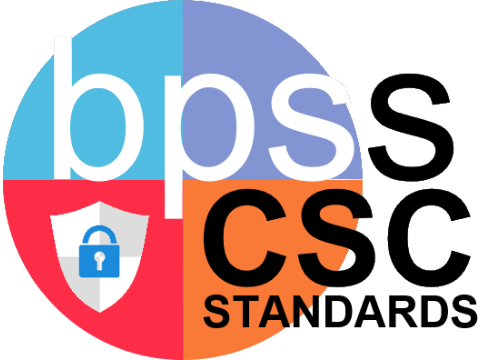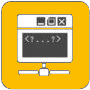Friday, March 31, 2023, 10:50 AM
Site: Learnbps
Course: BPSS (CSC) Computer Science and Cybersecurity (S-CSC)

#### CSC-02.CS# Computing in Society

## Sub-Concepts## Calculation Method for Concepts

Concepts are larger groups of related Sub-Concepts with standards. So the Concept is a calculation of all the related standards that calculate to the sub-concept. So click on the standard identifier below each Concept to access the learning targets and proficiency scales for each standard.

#### CSC-02.CT# Computation Thinking

## Sub-Concepts## Calculation Method for Concepts

Concepts are larger groups of related Sub-Concepts with standards. So the Concept is a calculation of all the related standards that calculate to the sub-concept. So click on the standard identifier below each Concept to access the learning targets and proficiency scales for each standard.

#### CSC-02.DC# Digital Citizenship

## Sub-Concepts## Calculation Method for Concepts

Concepts are larger groups of related Sub-Concepts with standards. So the Concept is a calculation of all the related standards that calculate to the sub-concept. So click on the standard identifier below each Concept to access the learning targets and proficiency scales for each standard.

#### CSC-02.IL# Information Literacy

## Sub-Concepts## Calculation Method for Concepts

Concepts are larger groups of related Sub-Concepts with standards. So the Concept is a calculation of all the related standards that calculate to the sub-concept. So click on the standard identifier below each Concept to access the learning targets and proficiency scales for each standard.

#### CSC-02.TS# Technology Systems

## Sub-Concepts## Calculation Method for Concepts

Concepts are larger groups of related Sub-Concepts with standards. So the Concept is a calculation of all the related standards that calculate to the sub-concept. So click on the standard identifier below each Concept to access the learning targets and proficiency scales for each standard.